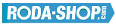# Remote ControlManufacturer Product Image Item Name Price### 629-2RS

Miniature Metric Series Chrome Steel Rubber Seals on Both sides Dimension d=9.00 Dimension D=26.00 Dimension B=8.00 Dinamic Load Rating=4575 Static Load Rating=1983 Weight=0.022gr. Speed limit Grease=28.0 Speed limit Oil=34.0
inside diameter = 9.00
outside diameter = 26.00
width = 8.00
€2.24### 629-2Z

Miniature Metric Series Chrome Steel Metallic Shields on both sides Dimension d=9.00 Dimension D=26.00 Dimension B=8.00 Dinamic Load Rating=4575 Static Load Rating=1983 Weight=0.022gr. Speed limit Grease=28.0 Speed limit Oil=34.0
inside diameter = 9.00
outside diameter = 26.00
width = 8.00
€2.24### 630/8-2RS

Miniature Metric Series Chrome Steel Rubber Seals on Both sides Dimension d=8.00 Dimension D=22.00 Dimension B=11.00 Dinamic Load Rating=3293 Static Load Rating=1379 Weight=0.019gr. Speed limit Grease=34.0 Speed limit Oil=40.0
inside diameter = 8.00
outside diameter = 22.00
width = 11.00
€2.24### 630/8-2Z

Miniature Metric Series Chrome Steel Metallic Shields on both sides Dimension d=8.00 Dimension D=22.00 Dimension B=11.00 Dinamic Load Rating=3293 Static Load Rating=1379 Weight=0.019gr. Speed limit Grease=34.0 Speed limit Oil=40.0
inside diameter = 8.00
outside diameter = 22.00
width = 11.00
€2.24### 633-2Z

Miniature Metric Series Chrome Steel Metallic Shields on both sides Dimension d=3.00 Dimension D=13.00 Dimension B=5.00 Dinamic Load Rating=1301 Static Load Rating=488 Weight=0.004gr. Speed limit Grease=40.0 Speed limit Oil=48.0
inside diameter = 3.00
outside diameter = 13.00
width = 5.00
€2.24### 634

Miniature Metric Series Chrome Steel Open(No Shields) Dimension d=4.00 Dimension D=16.00 Dimension B=5.00 Dinamic Load Rating=1340 Static Load Rating=523 Weight=0.006gr. Speed limit Grease=36.0 Speed limit Oil=43.0
inside diameter = 4.00
outside diameter = 16.00
width = 5.00
€2.24### 634-2RS

Miniature Metric Series Chrome Steel Rubber Seals on Both sides Dimension d=4.00 Dimension D=16.00 Dimension B=5.00 Dinamic Load Rating=1340 Static Load Rating=523 Weight=0.006gr. Speed limit Grease=36.0 Speed limit Oil=43.0
inside diameter = 4.00
outside diameter = 16.00
width = 5.00
€2.24### 634-2Z

Miniature Metric Series Chrome Steel Metallic Shields on both sides Dimension d=4.00 Dimension D=16.00 Dimension B=5.00 Dinamic Load Rating=1340 Static Load Rating=523 Weight=0.006gr. Speed limit Grease=36.0 Speed limit Oil=43.0
inside diameter = 4.00
outside diameter = 16.00
width = 5.00
€2.24### 635

Miniature Metric Series Chrome Steel Open(No Shields) Dimension d=5.00 Dimension D=19.00 Dimension B=6.00 Dinamic Load Rating=2336 Static Load Rating=896 Weight=0.008gr. Speed limit Grease=32.0 Speed limit Oil=40.0
inside diameter = 5.00
outside diameter = 19.00
width = 6.00
€2.24### 635-2RS

Miniature Metric Series Chrome Steel Rubber Seals on Both sides Dimension d=5.00 Dimension D=19.00 Dimension B=6.00 Dinamic Load Rating=2336 Static Load Rating=896 Weight=0.008gr. Speed limit Grease=32.0 Speed limit Oil=40.0
inside diameter = 5.00
outside diameter = 19.00
width = 6.00
€2.24